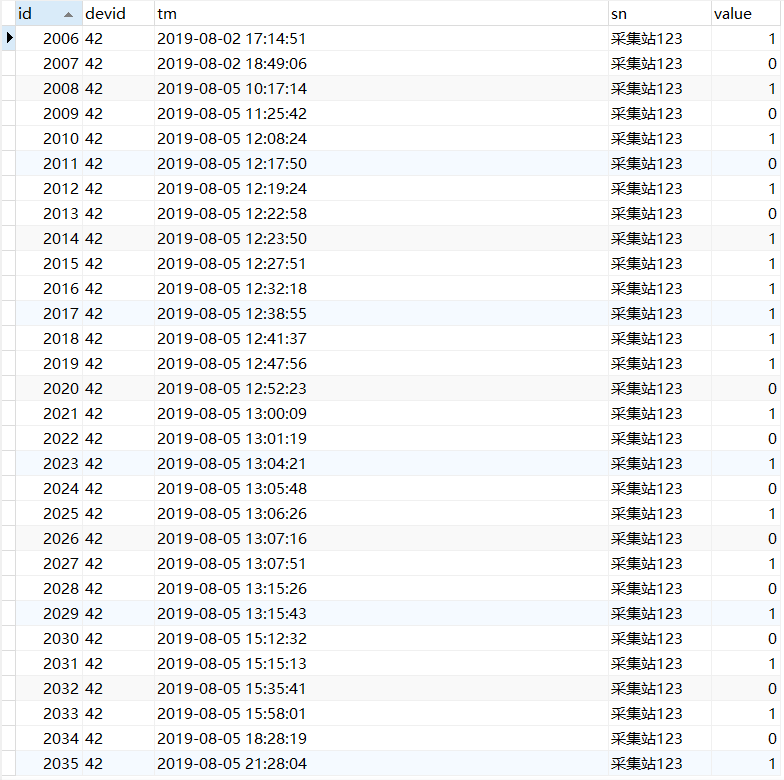mysql 数据查询问题，通过一个字段，以及时间将同一表内两条数据合并，也可能没有对应数据## 数据库如图

value是上下线记录1是上线0是下线，可能会有异常数据（有上线没有下线）求大神给个sql解决一哈，将上下线合并为一条

3个回答

SELECT C,TO_CHAR(WM_CONCAT(DATA)) FROM (
SELECT C,TO_CHAR(Q,'YYYYMMDD')||'上线到'||TO_CHAR(W,'YYYYMMDD')||'下线' DATA FROM (
SELECT C,MIN(Q) Q,W FROM(
SELECT C,Q,W FROM(
SELECT H.*
,ROW_NUMBER()OVER(PARTITION BY Q,C ORDER BY W ) RM
FROM (
SELECT *
FROM (SELECT A.ID C,A.DATA Q FROM UP A WHERE A.UP_DOWN=1 ORDER BY A.DATA ) A
LEFT JOIN (SELECT B.ID D,B.DATA W FROM UP B WHERE B.UP_DOWN=0 ) B
ON C=D WHERE Q<W
) H
)
WHERE RM=1
)GROUP BY C,W
ORDER BY Q
)
) GROUP BY C